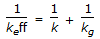# Chemical Engineering - Chemical Reaction Engineering

### Exercise :: Chemical Reaction Engineering - Section 3

46.

A first order gaseous phase reaction is catalysed by a non-porous solid. The kinetic rate constant and the external mass transfer co-efficients are k and kg respectively. The effective rate constant (keff) is given by

 A. keff = k + kg B. keff = (k+ kg)/2 C. keff = (kkg)1/2 D.Explanation:

No answer description available for this question. Let us discuss.

47.

Arrhenious equation represents graphically the variation between the __________ and temperature.

 A. rate of reaction B. frequency factor C. rate constant D. activation energy

Explanation:

No answer description available for this question. Let us discuss.

48.

__________ catalytic reaction is involved in the thermal cracking of gas oil.

 A. Homogeneous B. Homogeneous non C. Heterogeneous D. Heterogeneous non

Explanation:

No answer description available for this question. Let us discuss.

49.

Variables affecting the rate of homogeneous reactions are

 A. pressure and temperature only. B. temperature and composition only. C. pressure and composition only. D. pressure, temperature and composition.

Explanation:

No answer description available for this question. Let us discuss.

50.

A chemical reaction occurs when the energy of the reacting molecules is __________ the activation energy of the reaction.

 A. less than B. equal to C. more than D. equal to or more than